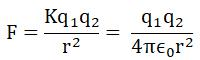Courses

# Test: Coulomb’S Law

## 10 Questions MCQ Test Physics Class 12 | Test: Coulomb’S Law

Description
This mock test of Test: Coulomb’S Law for Class 12 helps you for every Class 12 entrance exam. This contains 10 Multiple Choice Questions for Class 12 Test: Coulomb’S Law (mcq) to study with solutions a complete question bank. The solved questions answers in this Test: Coulomb’S Law quiz give you a good mix of easy questions and tough questions. Class 12 students definitely take this Test: Coulomb’S Law exercise for a better result in the exam. You can find other Test: Coulomb’S Law extra questions, long questions & short questions for Class 12 on EduRev as well by searching above.
QUESTION: 1

### Two insulated charged copper spheres A and B have their centers separated by a distance of 50 cm. What is the mutual force of electrostatic repulsion if the charge on each is 6.5×10−7 C? The radii of A and B are negligible compared to the distance of separation.

Solution: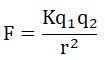QUESTION: 2

### Two charges 1C and -4C exists in air. What is the direction of force?

Solution:

Since the charges are unlike, the force will be attractive. Thus the force directs from 1C to -4C.

QUESTION: 3

### Ratio of the permittivity of medium to the permittivity of free space is known as

Solution: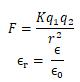Where ∈r is called the dielectric constant or relative permittivity of the medium

QUESTION: 4

An infinite number of charges, each equal to 4C, are placed along the x-axis at x = 1 m, x = 2 m, x = 4 m, x = 8 m and so on. The total force on a charge of 1C placed at the origin is

Solution: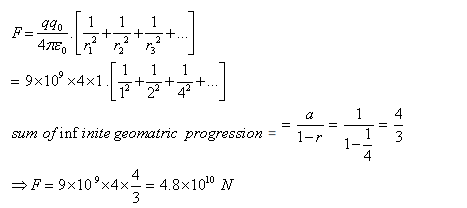QUESTION: 5

Two point charges 3μC and + 7μC repel each other with a force of 12 N. If each is given an additional charge -5 μC, what will be the new force between them?

Solution: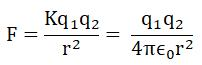QUESTION: 6

Electrostatic force is

Solution:

Electrostatics means charges at rest, or relatively at rest so this force can be applicable only when charge produce electric field which is constant which is possible only when charges at rest or relatively at rest.

QUESTION: 7

Two positive charges

Solution:

According to coulomb's law unlike charges always attract each other while like charges always repel each other so between two positive charges repulsion is working.

QUESTION: 8

Under the influence of the coulomb field of charge +Q, a charge −q is moving around it in an elliptical orbit. Find out the correct statement(s).

Solution:

Since the charge –q is moving in elliptical orbit so to make its motion stable the total angular momentum of the charge is constant since it experience a centripetal force from the charge +Q so it follow the motion as the motion of earth around sun.

QUESTION: 9

The force between two charges is 120 N. If the distance between the charges is doubled, the force will be

Solution: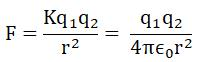QUESTION: 10

When the distance between two charged particle is halved, the force between them becomes

Solution: